# Solution assignment 03 Quadratic equations (abc-formula)

### Assignment 3

Solve: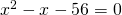### Solution

We can factorize the left-hand side: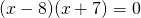and thus the solutions are: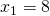or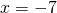We could get the same result by applying the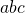-formula: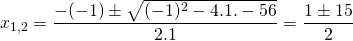0